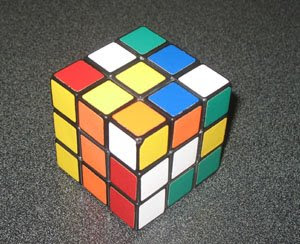## Wednesday, September 17, 2008

### 54

54 = 2 x 33.

54
is the smallest number that can be written as a sum of three squares in three ways: 54 = 12 + 22 + 72 = 22 + 52 + 52 = 32 + 32 + 62.

54 is the number of integer partitions of 19 into distinct parts.

A polygonal number can be represented as nested points arranged in the form of a regular polygon. 54 is a nonadecagonal (19-gonal) number: 1, 19, 54, 106, 175, . . .

54 is 110110 in base 2 and 66 in base 8.Sources:
Wikipedia; Number Gossip